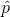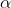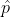Question

Solution :

Given that,

n = 2085

x = 355

Point estimate = sample proportion == x / n = 355/2089=0.170

1 -= 1-0.170 =0.83

At 98% confidence level the z is ,= 1 - 98% = 1 - 0.98 = 0.02/ 2 = 0.02/ 2 = 0.01

Z/2 = Z0.01 = 2.326 ( Using z table    )

Margin of error = E = Z/ 2 *(((* (1 -)) / n)

= 2.326 (((0.170*0.83) /2085 )

E = 0.019

A 98% confidence interval for population proportion p is ,- E < p <+ E

0.170-0.019 < p <0.170+ 0.019

0.151< p < 0.189

The 98% confidence interval for the population proportion p is : 0.151< p < 0.189

#### Earn Coins

Coins can be redeemed for fabulous gifts.

Similar Homework Help Questions